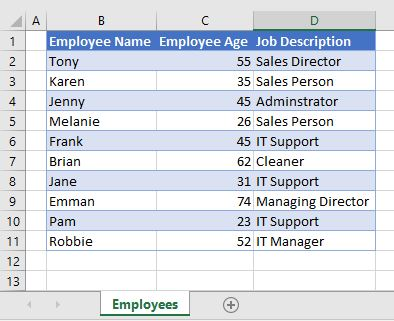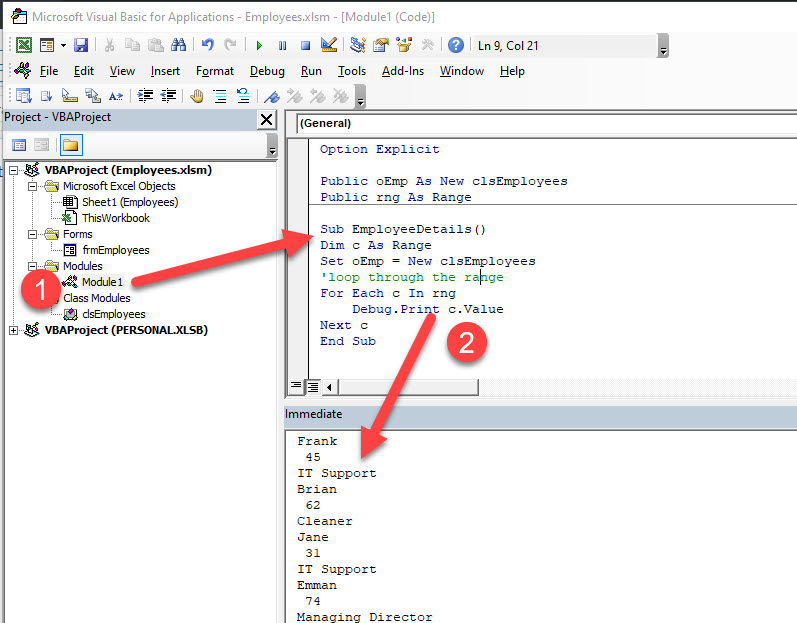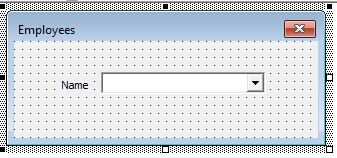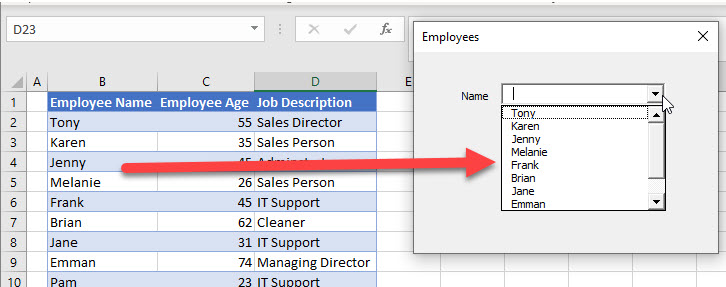# VBA Class Constructor

This tutorial will teach you about the Class Constructor in Class Modules in VBA.

When you create a Class Module in VBA, you can create a class Constructor that will run when the class is called.  This Constructor is called the Initialize Event in VBA.

## Initialize Event in a Class Module

Consider the worksheet below:In the VBA code, we can add a class module called clsEmployees to the VBA Project, and then we can create an Initialize Event which can set the Range object as the Range (“B2:D11”) in the Employees Sheet.

``````Private Sub Class_Initialize()
Set rng = Worksheets("Employees").Range("B2:D11")
End Sub``````

In a standard module, we can then call this class and we are then able to run through all the details contained in the range.In the example above, 1) the EmployeeDetails sub-procedure is created within a new module. It creates a new instance of clsEmployees. In doing so, the initialize event in the class is fired, which in turn sets the Range object.

We can then 2) loop through the Range Object using the For Each…Next Loop to Debug.Print each value in the Range as shown in the immediate window below.

## Initialize Event in a User Form

Userforms are essentially class modules.  Therefore they too have an initialize event and therefore a Class Constructor.

Take the following form for example.  It contains a ComboBox called cboEmployees.In the Initialize Event of this form, we can populate the combo box as follows:This is done using the following code:

``````Private Sub UserForm_Initialize()
Dim c As Range
Dim rng As Range
Set rng = Worksheets("Employees").Range("B2:D11")
For Each c In rng
If c.Column = 2 Then
End If
Next c
End Sub``````

When the form is first opened, the Initalize event (Class Constructor) will fire.  This will populate the variable rng with the Range (“B2:D12”).  We will then declare another range object (c) which will be populated when we loop through the rng.  If the column is column B (ie 2), then the name of the employee will be added to the combo list.

Stop searching for VBA code online. Learn more about AutoMacro - A VBA Code Builder that allows beginners to code procedures from scratch with minimal coding knowledge and with many time-saving features for all users!